## A Glimpse of Infinity In the Swirling of Light

Infinite mathematics, long thought to be impossible to observe, manifests in optical vortices.

#### MacroscopeMathematicsPhysics

If the doors of perception were cleansed every thing would appear to man as it is, Infinite. For man has closed himself up, till he sees all things thro’ narrow chinks of his cavern.
—William Blake, “The Marriage of Heaven and Hell” (1793)

What is the meaning of the word infinity? Metaphorically, it has always represented something unattainable, something that does not exist in our mundane reality: always larger than the largest thing imaginable. This is also true in modern physics, where early concepts of a universe infinite in both space and time were replaced by a rapidly expanding but finite cosmos birthed in the Big Bang. Nothing in physics, it would seem, is infinite after all.

Undeterred, mathematicians of the 19th century studied in detail the properties of infinite sets of objects. This effort, led by the brilliant Georg Cantor (1845–1918), is now known as the theory of transfinite numbers and demonstrated many surprising and nonintuitive features of infinite sets. But the strangeness of infinite mathematics seemed to reinforce the idea that infinity is something outside of human observation and understanding.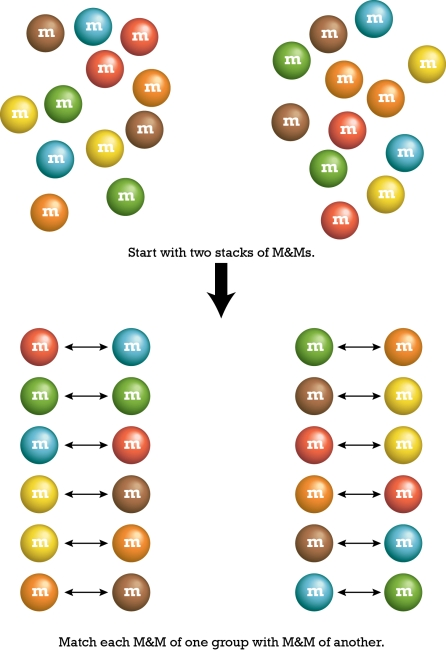This specter of unattainability has changed over the past year, as two papers—including one I wrote—demonstrated that infinite mathematics can be realized in optical systems possessing swirling vortices of light. In particular, both demonstrations showed how particular systems mimic exactly a paradoxical thought experiment known as Hilbert’s Hotel, in which the occupancy of a hotel consisting of an infinite number of rooms shows very strange behavior. These examples seem to be the first ever showing that infinite mathematics not only manifests in some real world systems, but is essential in explaining them.

To understand these results, we begin by discussing the size of infinity. Is infinity a “one-size-fits-all” concept, or are some infinities bigger than others? For example, is the set of natural numbers, 1,2,3,4,5,…, larger than the set of even natural numbers, 2,4,6,8,…? At first glance, most people would say “yes,” but to properly answer the question we need a rigorous way to compare the sizes of infinite sets, because we simply can’t count them.

Cantor introduced the idea of comparing the sizes of sets by establishing a one-to-one correspondence between them. To see what this means, imagine that we have two large piles of M&Ms , and want to see if they are the same size. The most efficient way to do this is match up M&Ms from each pile, as shown in the figure (above on the right). If every M&M of one pile can be matched to an M&M of the other pile, with none unaccounted for, the size of the piles is the same.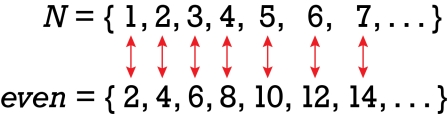This method can be extended to infinite sets, as well. For instance, we can show that the size of the set of natural numbers is the same as the size of the set of even numbers, because we can line them up in a one-to-one correspondence, as well.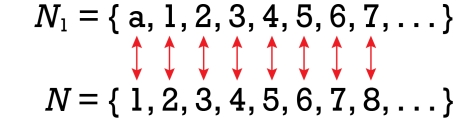We can also make quantitative the statement that “infinity plus one equals infinity” for the natural numbers, by observing that the set a,1,2,3,4,… can be put into one-to-one correspondence with the natural numbers alone.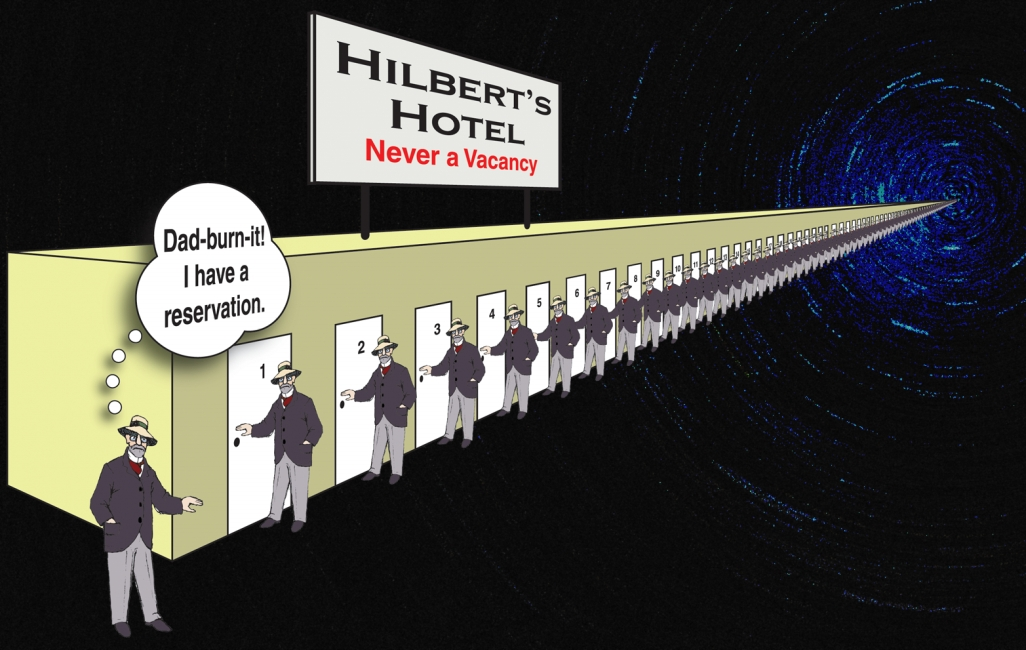The strangeness of this was summarized by the great mathematician David Hilbert in the 1920s, in the paradoxical “Hilbert’s Hotel”: We imagine a hotel with an infinite number of rooms, labeled with the natural numbers, and imagine that each room is occupied, so the hotel has no vacancies.

But applying the same idea of one-to-one correspondence, we can ask each guest to move to the next-highest room in the hotel. Because we have a countably infinite number of rooms, every guest will be able to move over, leaving Room 1 vacant for the new guest.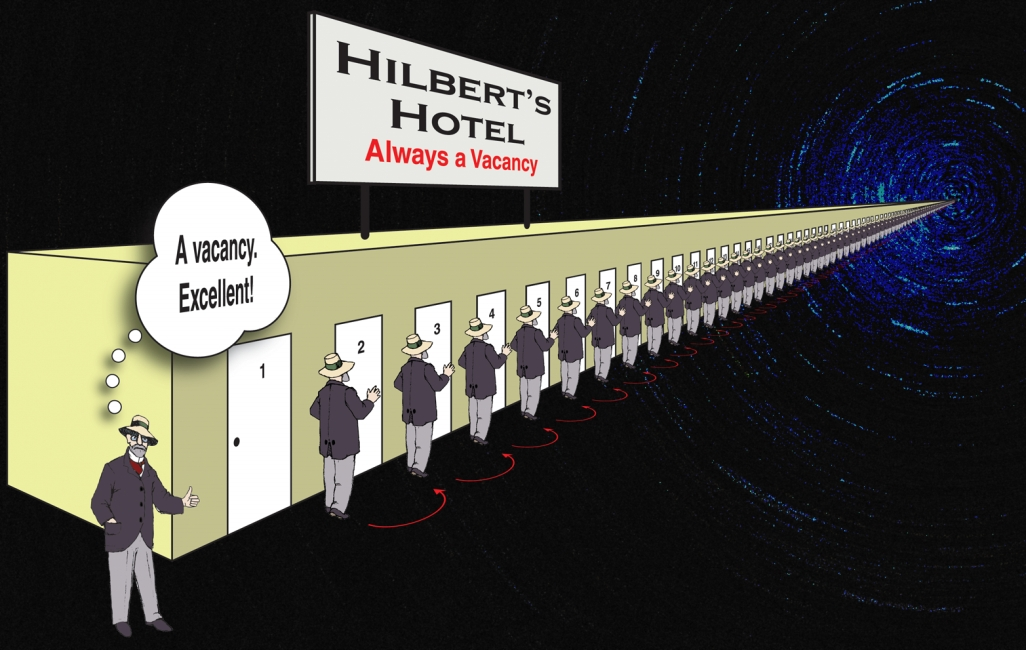This process can be repeated indefinitely, allowing any number of new guests to get rooms. Hilbert’s Hotel, therefore, is simultaneously completely filled and also has unlimited vacancies!

Cantor also proved that some infinities are larger than others. The size of the set of countable numbers (1,2,3,4,…) is smaller than the infinite set of all decimal numbers on the number line between zero and 1, which includes fractions such as ½ and ¾ as well as irrational numbers such as π/4.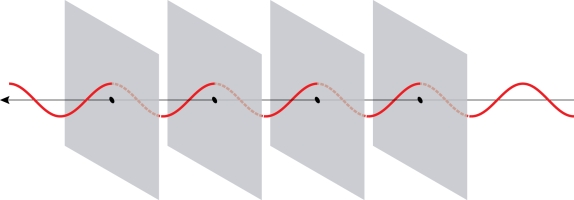Even without considering the larger infinities, it would seem at first glance that the strange mathematics of countably infinite sets would never make an appearance in a physical system, because it would result in curious paradoxes like Hilbert’s Hotel. But this turns out not to be true—when vortices are present in beams of light, strange things can happen!

Most people are somewhat familiar with the idea that light has wave properties. Much like the ripples on a pond, a light wave has a series of up-and-down wiggles as it travels. The simplest sort of wave one can visualize is called a plane wave, as pictured above.

In this picture, the wave travels to the left, and in every plane perpendicular to its motion it is at the same stage of “wiggling.” The planes where the wave is “up” are usually referred to as wavefronts, and we can get a feeling for the overall behavior of the wave by looking at the wavefronts alone.It is also possible, however, to produce a beam of light in which the wavefronts are joined together, forming a helical structure with the shape of a screw or drill bit (see figure above). The motion of the wave to the left is equivalent to it spinning: In addition to the wave propagating to the left, it has circular motion around its central axis. For this reason, it is known as an optical vortex.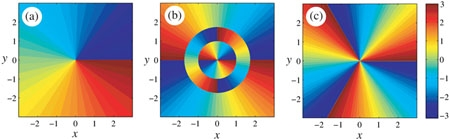When we are illustrating the vortices of light, it is more convenient to draw the phase of light in a cross-section of a light beam. For our purposes, we can imagine looking at the beam head-on from the left side of the above picture: the phase is, in essence, the topographical map of a section of the wavefront from that perspective. The cross-sections of several optical vortex beams are shown above; the color indicates the “height” of the wavefront. The first picture shows what the vortex in the above picture looks like from this new perspective; as one goes in a counter-clockwise path around the center, the ramp increases from the lowest value (blue) to the highest value (red). The other figures show vortices of higher order, where the order is the total number of blue-to-red ramps in a single cross-section; in the third example, it is of negative order, as it goes red to blue in the counter-clockwise direction.

A light wave can have many vortices nested in it, in different parts of the wave’s cross-section; I often like to describe a wave with many vortices as looking like a parking deck, with many ramps going up and down between levels at different locations.

There are two properties of optical vortices important to us here. First, because the wave itself must be smooth, a vortex can only ramp up or down by an integer number of “levels” of a parking deck: We can’t have a parking garage where the ramp goes up or down half a level. The number of red-to-blue ramps the vortex has in a counter-clockwise direction is known as the topological charge of the vortex, and must be an integer number: … –3, –2, –1,0,1,2,3,…. For a beam with a single vortex, as in the pictures above, the topological charge is the same as the order of the beam. Second, it can be shown that topological charge is generally a conserved quantity. If we distort an optical beam, we find that the vortices in the beam will move around but not disappear unless they annihilate with a vortex of opposite charge. The situation is strikingly similar to the behavior of elementary particles in high-energy physics: Electrons with negative charge will only disappear if they annihilate with their antiparticles, positrons, with positive charge.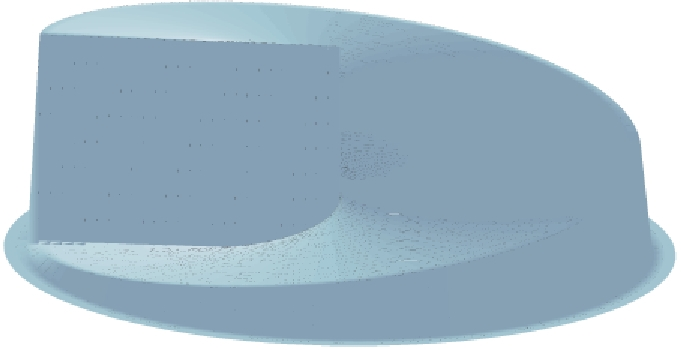This conservation of topological charge raises the question: How does one get an unbalanced vortex in a beam of light? Experimentally, one can do it by passing a light beam through a spiral phase plate, such as the one illustrated on the right. The plate is made of a piece of glass that is fashioned to have a ramp shape. As a plane wave, coming from below, passes through the plate, the wavefronts get slowed down in the glass in proportion to the thickness of the plate at that point. If the plate material and thickness are chosen carefully, one can arrange it so that the plate produces a beam with a perfect integer topological charge.

But this raises an interesting question: What happens if the spiral phase plate is designed to make a noninteger topological charge beam? There is nothing preventing this; to make a plate that is effectively charge ½, we just need to make a plate with ½ the thickness of a charge 1 plate. But a charge ½ vortex cannot exist, so something unusual must happen.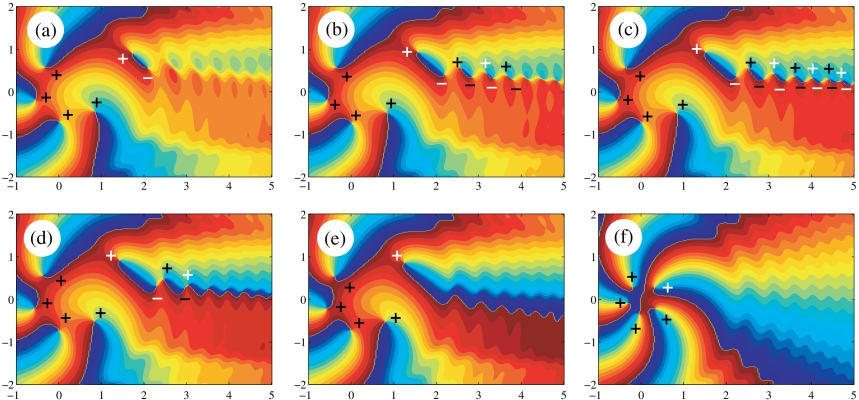This problem was explored both theoretically and experimentally back in 2004. The theoretical work was done by Michael Berry, who explored what happens to a vortex beam when the plate’s “charge” is continuously increased between two integer values. For example, in the image below we show the evolution of the vortices in an optical field that has the plate charge gradually increased from 3 to 4.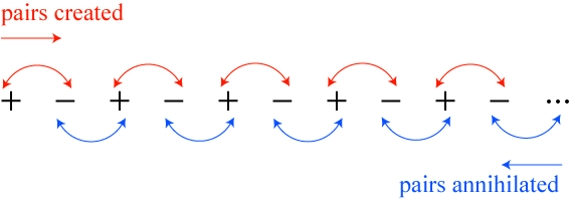When the charge of the plate, let us refer to it as t, is equal to 4, we have a single third-order vortex. As the charge of the plate is increased and approaches t = 4.5, a peculiar thing happens: Pairs of vortices are created, starting near the center of the field, and gradually appearing further and further away to the right. It can be shown that at t = 4.5, there are an infinite number of vortex pairs. Then, as t is increased above 4.5, these vortex pairs begin to annihilate, starting from infinity and ending near the center of the field. However, each negative charge annihilates with its right neighbor, instead of the left neighbor with which it was created. The net result is that there is a single extra unbalanced positive charge left over!

It is this behavior that perfectly mimics Hilbert’s Hotel! If we imagine the positive charges as rooms, and the negative charges as guests, then the transition from a field with charge 3 to a field with charge 4 happens as follows. Every “guest” (negative charge) steps out of their “room” (positive charge), and moves into the room to the right. The net result is a single additional unoccupied room (positive charge).

What is truly remarkable about this effect is that the system uses the strange mathematics of infinity to get around the conservation of topological charge. Because a charge cannot be created from nothing, the system instead creates an infinite number of charge pairs. Then, using the strange mathematics of infinity that allows “infinity plus one equals infinity,” a single new positive charge is created. We find not only an optical system that uses weird infinite addition, but in fact requires it in order to create a vortex beam!

This relationship between infinite mathematics and optical vortices seems to have been previously unappreciated, at least in scientific publications. I happened across it while reviewing the properties of such “fractional vortex systems,” and have written up these observations for the journal Optica.

It is very important to note, however, that one never actually sees the infinite chain of vortices when t = 3.5. The theoretical calculation was done for a light wave of infinite transverse extent, while any realistic wave will have a small cross-section. In practice, then, the infinite chain of vortex pairs gets lost in the darkness of the edge of the beam; however, we may say that we see the “signature” of infinity in this process. And, experimentally, the dance of vortices described here was also confirmed back in 2004.

This is not the only optical system to exhibit infinite mathematics; in fact, it is not even the first observed! At almost the same time I was noting the connection between optical vortices and fractional spiral phase plates, an international collaboration of researchers submitted their work on a system that mimics the relationship between the natural numbers and the even numbers discussed above.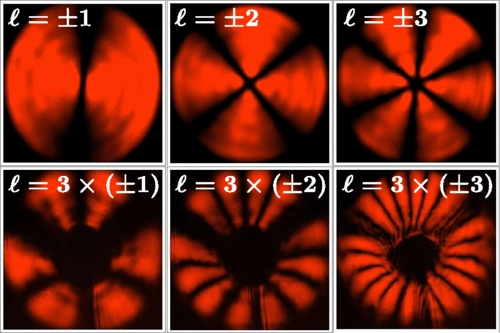In this research, the collaboration demonstrated an optical system that takes a vortex beam with topological charge t and transforms it into a beam with charge 2t, or 3t. This, in essence, mimics the more general Hilbert’s Hotel scenario in which every guest in room N moves to the room 3N, allowing an infinite number of new guests simultaneously!

I personally find all of these results quite astounding. Although we have traditionally thought of the mathematics of infinity to be more or less an elegant thought experiment, it turns out that it plays a key role in at least two optical systems! I fully imagine that other connections between optical vortices and infinite mathematics will be uncovered. Will practical results come from these connections? At this point, it is impossible to say.

#### Infinity Mirrors and Beyond

If you’re skeptical about all of these results, it should be noted that you yourself have almost certainly seen another optical system that exhibits a peculiar infinite mathematical paradox. The system in question is an infinity mirror, two parallel mirrors placed facing each other, in which one can see an infinite number of images of oneself, stretching out into infinity.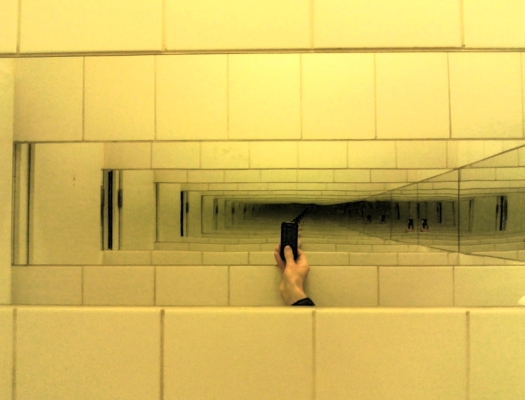The width of each image is inversely related to its apparent distance: If the apparent distance is d, the image width is 1/d. A similar argument applies to the width. But this means that the area of the image is inversely related to the square of the distance, 1/d2. Curiously, one can show that the total area of all the images, taken to infinity, is finite, but the combined width of all the images is infinite!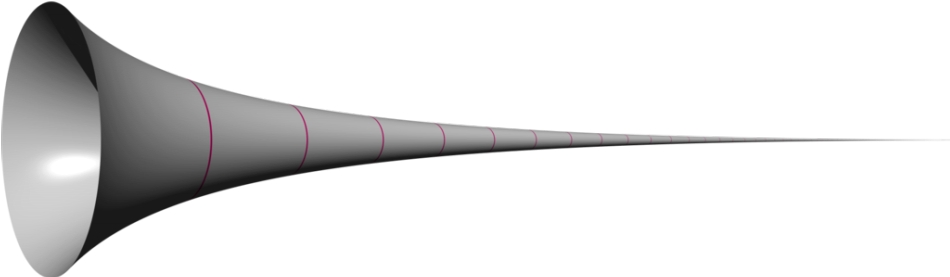This is a paradoxical observation and is closely related to a mathematical object known as Gabriel’s Horn. The horn, which stretches off infinitely to the right, can be shown to have a finite volume but an infinite surface area. This leads to the very strange possibility that Gabriel’s horn could be filled with a finite amount of paint, but that paint could not be used to coat the outside of the horn, no matter how thin a coat is used.

Just as in the vortex example above, though, the infinite set of images is never directly observed. As the images get further away, they become too dim to see and they tend to drift out of sight on the side of the mirror. Nature is good at hiding its infinities, it seems. I find this one of the interesting things about studying infinity in optical systems. It remains to be seen whether these surprising results will lead to even more insight into the nature of light and physics.

×